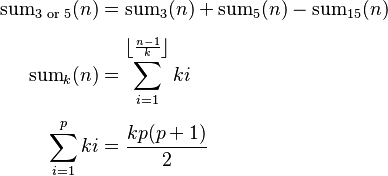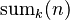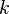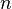# Project Euler facts for kids

Kids Encyclopedia Facts
 Type of siteProblem Solving Website Colin Hughes projecteuler.net No Free October 5, 2001

Project Euler (named after Leonhard Euler) is a website dedicated to a series of computational problems intended to be solved with computer programs. The project attracts adults and students interested in mathematics and computer programming. Since its creation in 2001 by Colin Hughes, Project Euler has gained notability and popularity worldwide. It includes over 700 problems, with a new one added once every one or two weeks. Problems are of varying difficulty, but each is solvable in less than a minute of CPU time using an efficient algorithm on a modestly powered computer. As of 5 April  2020, Project Euler has more than 1,000,000 users, from all over the world, who have solved at least one problem.

## Features of the site

A forum specific to each question may be viewed after the user has correctly answered the given question. Problems can be sorted on ID, number solved and difficulty. Participants can track their progress through achievement levels based on the number of problems solved. A new level is reached for every 25 problems solved. Special awards exist for solving special combinations of problems. For instance, there is an award for solving fifty prime numbered problems. A special "Eulerians" level exists to track achievement based on the fastest fifty solvers of recent problems so that newer members can compete without solving older problems.

## Example problem and solutions

The first Project Euler problem is

If we list all the natural numbers below 10 that are multiples of 3 or 5, we get 3, 5, 6 and 9. The sum of these multiples is 23.

Find the sum of all the multiples of 3 or 5 below 1000.

Though this problem is much simpler than the typical problem, it serves to illustrate the potential difference that an efficient algorithm makes. The brute-force algorithm examines every natural number less than 1000 and keeps a running sum of those meeting the criteria. This method is simple to implement, as shown by the following pseudocode:

total := 0
for NUM from 1 through 999 do
if NUM mod 3 = 0 or NUM mod 5 = 0 then
total := total + NUM
return total


For harder problems, it becomes increasingly important to find an efficient algorithm. For this problem, we can reduce 1000 operations to a few by using the inclusion–exclusion principle and a closed-form summation formula.\begin{align} \mathrm{sum}_{\text {3 or 5}}(n) & = \mathrm{sum}_3(n) + \mathrm{sum}_5(n) - \mathrm{sum}_{15}(n) \\[4pt] \mathrm{sum}_k(n) & = \sum_{i=1}^{\left \lfloor \frac{n-1}{k} \right \rfloor} ki \\[4pt] \sum_{i=1}^p ki & = \frac{kp(p+1)}{2} \end{align}

Here,$\mathrm{sum}_k(n)$ denotes the sum of multiples of$k$ below$n$. In big O notation, the brute-force algorithm is O(n) and the efficient algorithm is O(1) (assuming constant time arithmetic operations).Project Euler Facts for Kids. Kiddle Encyclopedia.